Objections Maths with AGM Pressure

 This page describes an analysis of the behaviour of anti-gravity matter around a small central normal matter object when AGM Pressure is involved.  This analysis is much simplified by the observation described in Investigation that for small scale phenomena anti-gravity between anti-gravity matter particles can be ignored.  In the analysis we assume that Gaa = 0.   Definitions and Assumptions Gravitational constant for normal matter to normal matter attraction = Gnn = conventional G Gravitational constant for normal matter to anti-gravity matter repulsion = Gna Gravitational constant for anti-gravity matter to anti-gravity matter repulsion = Gaa = 0 The background density of anti-gravity matter = Dab The AGM Exclusion Density = Dnx The background pressure of anti-gravity matter = Pab Assume monatomic adiabatic expansion where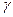= 5/3What’s the relationship between anti-gravity matter pressure and radius?   Assume anti-gravity matter is arranged spherically symmetrically around a central normal matter object of mass M. Radius variable = r Anti-gravity matter density at any radius  =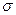Anti-gravity matter pressure at any radius = p For any thin concentric spherical shell weight (outwards) is supported by change of pressure.   1)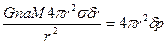The equation for adiabatic expansion of anti-gravity matter as pressure decreases   2)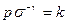where k is a constant   Therefore   3)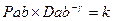But from 1) and 2)   4)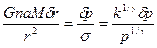Integrate   5)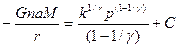When r =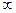p = Pab and from 3)   6)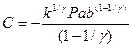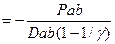At the AGM Boundary let r = R,  p = 0 and from 5) and 6)   7)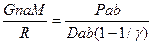8)      R =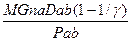and 9)      AGM Exclusion Density = Dnx =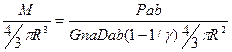and therefore   10)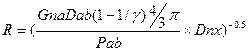Therefore from 8) the radius of the AGM Boundary is proportional to M, and from 10) inversely proportional to the square root of AGM Exclusion Density.

© Copyright Tim E Simmons 2010 to 2015. Last updated 27th July 2015.  Major changes are logged in AGM Change Log.